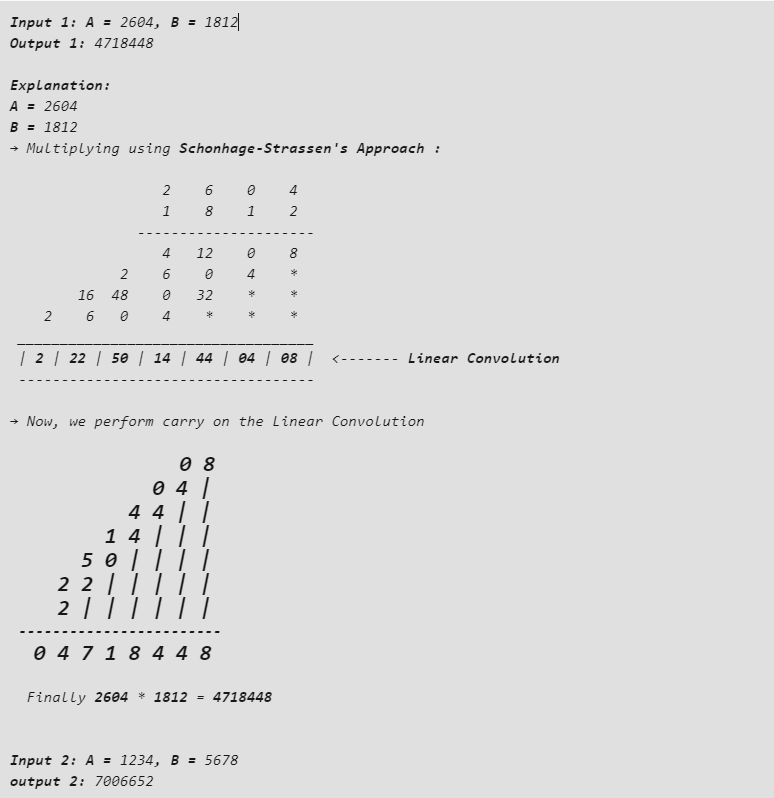Open in App
Not now

# Java Program to Implement the Schonhage-Strassen Algorithm for Multiplication of Two Numbers

• Last Updated : 12 Sep, 2022

Schonhage-Strassen algorithm is one of the fastest ways of multiplying very large integer values (30000 to 150000 decimal digits). This algorithm was developed by Arnold Schönhage and Volker Strassen. Though the Furer’s algorithm is faster than that of Schonhage-Strassen’s algorithm, there are no practical applications for it, except Galactic algorithms (probably not used for any data present on the earth). So, Schonhage-Strassen’s algorithm is considered the best for multiplying large integer values.

In this approach of multiplication, the two integers are first multiplied without performing carry. The result set then obtained is called Acyclic Convolution or Linear Convolution. Then we perform carry on the individual columns of the Linear Convolution.

Examples:## Java

 `// Java program to implement Schonhage-Strassen's` `// Multiplication Algorithm` `import` `java.io.*;`   `class` `GFG {` `    ``// two class level variables to` `    ``// store the given values` `    ``static` `long` `a, b;` `    ``// class level array to store the LinearConvolution` `    ``static` `int``[] linearConvolution;` `    ``// an integer variable to determine` `    ``// the length of the LinearConvolution` `    ``static` `int` `length;`   `    ``// to count the no.of digits in each of` `    ``// the given values a and b` `    ``static` `int` `countDigits(``long` `num)` `    ``{` `        ``// an integer variable initialized to` `        ``// 0 to store the no.of digits` `        ``int` `count = ``0``;` `        ``// as long as the number is` `        ``// greater than 0, divide it by 10` `        ``// and increment the count` `        ``while` `(num > ``0``) {` `            ``num /= ``10``;` `            ``count++;` `        ``}` `        ``// return the count when number becomes 0` `        ``return` `count;` `    ``}`   `    ``// to perform schonhage-Strassen's Multiplication` `    ``static` `void` `schonhageStrassenMultiplication()` `    ``{` `        ``// first find the LinearConvolution` `        ``findLinearConvolution();` `        ``// Then perform carry on it` `        ``performCarry();` `    ``}`   `    ``// to find LinearConvolution` `    ``static` `void` `findLinearConvolution()` `    ``{` `        ``// no.of digits in first number (a)` `        ``int` `aDigits = countDigits(a);` `        ``// no.of digits in second number (b)` `        ``int` `bDigits = countDigits(b);` `        ``// a temporary variable to store the value of a` `        ``long` `temp = a;` `        ``// length of the LinearConvolution is` `        ``// 1 less than the no.of Digits in a +` `        ``// no.of digits in b` `        ``length = aDigits + bDigits - ``1``;` `        ``linearConvolution = ``new` `int``[length];` `        ``for` `(``int` `i = ``0``; i < aDigits; i++, b /= ``10``) {` `            ``a = temp;` `            ``for` `(``int` `j = ``0``; j < bDigits; j++, a /= ``10``) {` `                ``// multiply the current digit of a with` `                ``// current digit of b and store in` `                ``// LinearConvolution` `                ``linearConvolution[i + j]` `                    ``+= (b % ``10``) * (a % ``10``);` `            ``}` `        ``}` `        ``System.out.print(``"The Linear Convolution is: [ "``);` `        ``for` `(``int` `i = length - ``1``; i >= ``0``; i--) {` `            ``// print the LinearConvolution array` `            ``System.out.print(linearConvolution[i] + ``"  "``);` `        ``}` `        ``System.out.println(``"]"``);` `    ``}`   `    ``// to perform carry on the obtained LinearConvolution` `    ``static` `void` `performCarry()` `    ``{` `        ``// initialize product to 0` `        ``long` `product = ``0``;` `        ``int` `carry = ``0``, base = ``1``;` `        ``// for every value in the LinearConvolution` `        ``for` `(``int` `i = ``0``; i < length; i++) {` `            ``linearConvolution[i] += carry;` `            ``// add the product of base and units digit of` `            ``// LinearConvolution[i] to the product` `            ``product` `                ``= product` `                  ``+ (base * (linearConvolution[i] % ``10``));` `            ``// now LinearConvolution[i]/10` `            ``// will become the carry` `            ``carry = linearConvolution[i] / ``10``;` `            ``base *= ``10``;` `        ``}` `        ``System.out.println(``"\nThe Product is : "` `+ product);` `    ``}`   `    ``// Driver method` `    ``public` `static` `void` `main(String[] args)` `    ``{` `        ``// initialize the two declared class variables with` `        ``// the desired values` `        ``a = ``2604``;` `        ``b = ``1812``;` `        ``// call schonhageStrassenMultiplication() method` `        ``schonhageStrassenMultiplication();` `    ``}` `}`

Output

```The Linear Convolution is: [ 2  22  50  14  44  4  8  ]

The Product is : 4718448```

Time Complexity: O(nlogn)

Auxiliary Space: O(n) because it is using extra space for array linearConvolution

My Personal Notes arrow_drop_up
Related Articles